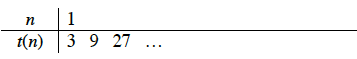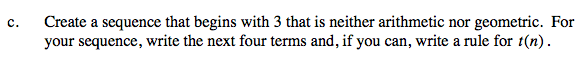Home > A2C > Chapter 2 > Lesson 2.2.1 > Problem2-128

2-128.
1. Consider the sequence 3, 9, … Homework Help ✎

1. Assuming that the sequence is arithmetic with t(1) as the first term, find the next four terms of the sequence and then write a rule for t(n).

2. Assuming that the sequence is geometric with t(1) as the first term, find the next four terms of the sequence and then write a rule for t(n).

3. Create a sequence that begins with 3 that is neither arithmetic nor geometric. For your sequence, write the next four terms and, if you can, write a rule for t(n).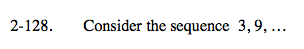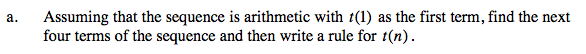Refer to the Math Notes boxes in Lessons 2.1.6 and 2.1.8, shown below.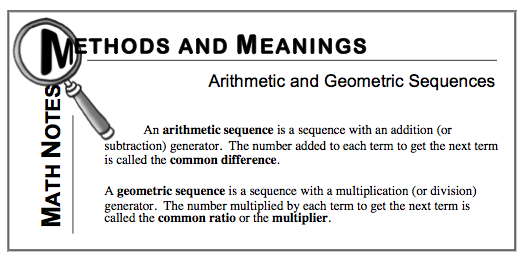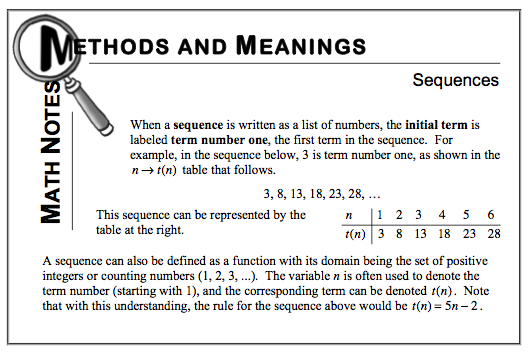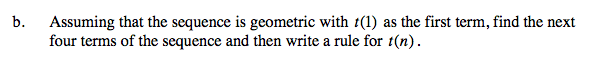Try copying and completing the table below: